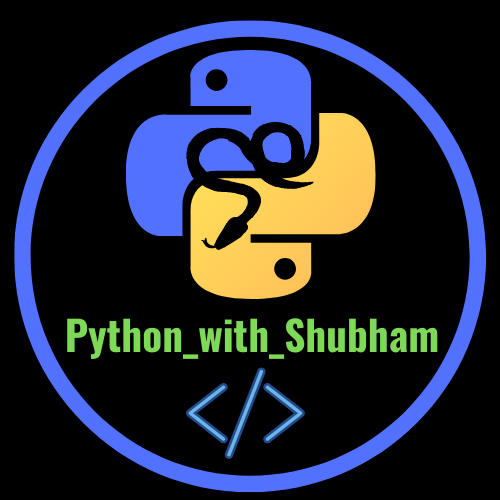# Python Programmingpython_with_shubham
June 13

Hello python lovers

### Introduction :-

Python is a General Purpose, Object-Oriented, Interpreted and High level Programming Language which is developed by Guido Van Rossum in 1989 and it is first released in February, 1991. Python is developed in Netherlands. The name of this Language is taken from “Monty Python's Flying Circus”. Python is written in C Programming Language

### Python-IDLE :-

IDLE is stand for Integrated Development and Learning Environment . It is an integrated development environment (IDE) for Python.

### Variables in Python:-

Variables are names given to data that we need to store and manipulate. A variable name in python only contains alphabet(a-z, A-Z), Number and underscore(_).

var = 0  (Correct)

var_1 = 3 (Correct)

var2 = "rohan"  (correct)

3var = "mohit" (wrong because it cannot contain number at the begining)

### Data Types in Python:- In Python mainly we have 3 types of Data Types-

1. Int - 2, 6, 84, 95

2. Float - 2.0, 5.4, 6.3, 8.0

3. String - "a", 'hello', "Python"

### Operators in Python:-

In Python we have following operators :-

### 1. Python Arithmetic Operators-

 Operator Name + Addition - subtraction * multiplication / Division % Modulus ** Exponentiation // Floor division

### 2. Python Comparison Operators-

 Operator Name == Equal != Not equal > Greater than < Less than >= Greater than or equal to <= Lesser than or equal to

### 3. Python Bitwise Operators-

 Operator Name & AND | OR ^ XOR ~ NOT << Zero fill left shift >> Signed right shift

### 4. Python Assignment Operators-

 Operator Example = x = 6 += x += 2 -= x -= 3 *= x *= 6 /= x /= 5 %= x%=1 //= x//=4 **= x**=8 &= x&=9 |= x|=4 ^= x^=3 >>= x>>=5 <<= x<<=1

### 5. Python Logical Operators-

 Operator Description and returns true if both statements are true or returns true if one of the statements is true not reverse the result. ex:- returns false if the result is true

### 6. Python Identity Operators-

 Operator Description is Returns True if both variables are the same object is not Returns True if both variables are not the same object

### 7. Python Membership Operators-

 operator description in Returns True if a sequence with the specified value is present in the object not in Returns True if a sequence with the specified value is not present in the object

***
##### Note:-  In the Next post we will discuss About Python if-else and loops.

+_+_+_+_+_+_+_+_+_+_+_+_+_+_

## More from the author### Python Programming